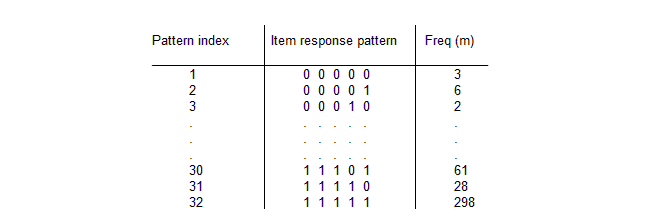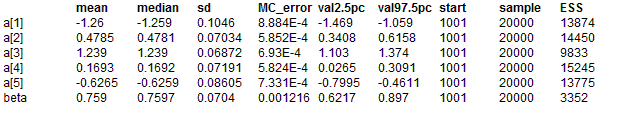#### LSAT: item response

Section 6 of the Law School Aptitude Test (LSAT) is a 5-item multiple choice test; students score 1 on each item for the correct answer and 0 otherwise, giving R = 32 possible response patterns.Boch and Lieberman (1970) present data on LSAT for N = 1000 students, part of which is shown below.The above data may be analysed using the one-parameter Rasch model (see Andersen (1980), pp.253-254; Boch and Aitkin (1981)). The probability pjk that student j responds correctly to item k is assumed to follow a logistic function parameterized by an `item difficulty' or threshold parameter αk and a latent variable θj representing the student's underlying ability. The ability parameters are assumed to have a Normal distribution in the population of students. That is:

logit(pjk) = θj - αk, j = 1,...,1000; k = 1,...,5

θj ~ Normal(0, τ)

The above model is equivalent to the following random effects logistic regression:

logit(pjk) = βθj - αk, j = 1,...,1000; k = 1,...,5

θj ~ Normal(0, 1)

where β corresponds to the scale parameter (β2 = τ) of the latent ability distribution. We assume a half-normal distribution with small precision for β; this represents vague prior information but constrains β to be positive. Standard vague normal priors are assumed for the αk's. Note that the location of the αk's depend upon the mean of the prior distribution for θj which we have arbitrarily fixed to be zero. Alternatively, Boch and Aitkin ensure identifiability by imposing a sum-to-zero constraint on the αk's. Hence we calculate ak = αk - αbar to enable comparision of the BUGS posterior parameter estimates with the Boch and Aitkin marginal maximum likelihood estimates.
BUGS language for LSAT model

model
{
# Calculate individual (binary) responses to each test from multinomial data
for (j in 1 : culm) {
for (k in 1 : T) {
r[j, k] <- response[1, k]
}
}
for (i in 2 : R) {
for (j in culm[i - 1] + 1 : culm[i]) {
for (k in 1 : T) {
r[j, k] <- response[i, k]
}
}
}
# Rasch model
for (j in 1 : N) {
for (k in 1 : T) {
logit(p[j, k]) <- beta * theta[j] - alpha[k]
r[j, k] ~ dbern(p[j, k])
}
theta[j] ~ dnorm(0, 1)
}
# Priors
for (k in 1 : T) {
alpha[k] ~ dnorm(0, 0.0001)
a[k] <- alpha[k] - mean(alpha[])
}
beta ~ dunif(0, 1000)
}

Note that the data are read into BUGS in the original multinomial format to economize on space and effort. The 5 times 1000 individual binary responses for each item and student are then created within BUGS using the index variable culm (read in from the data file), where culm[i] = cumulative number of students recording response patterns 1, 2, ..., i; i <= R.

NB when generating initial values do not check the fix founder box in the Specification Tool. If you do all the theta will be set to zero and beta will go to la-la land.
##### Data
``` list(N = 1000, R = 32, T = 5,      culm = c(3, 9, 11, 22, 23, 24, 27, 31, 32, 40, 40, 56, 56, 59, 61, 76, 86, 115, 129, 210, 213, 241, 256, 336, 352, 408,               429, 602, 613, 674, 702, 1000),   response = structure(.Data = c(                     0, 0, 0, 0, 0,                0, 0, 0, 0, 1,                0, 0, 0, 1, 0,                0, 0, 0, 1, 1,                0, 0, 1, 0, 0,                0, 0, 1, 0, 1,                0, 0, 1, 1, 0,                0, 0, 1, 1, 1,                0, 1, 0, 0, 0,                0, 1, 0, 0, 1,                0, 1, 0, 1, 0,                0, 1, 0, 1, 1,                0, 1, 1, 0, 0,                0, 1, 1, 0, 1,                0, 1, 1, 1, 0,                0, 1, 1, 1, 1,                1, 0, 0, 0, 0,                1, 0, 0, 0, 1,                1, 0, 0, 1, 0,                1, 0, 0, 1, 1,                1, 0, 1, 0, 0,                1, 0, 1, 0, 1,                1, 0, 1, 1, 0,                1, 0, 1, 1, 1,                1, 1, 0, 0, 0,                1, 1, 0, 0, 1,                1, 1, 0, 1, 0,                1, 1, 0, 1, 1,                1, 1, 1, 0, 0,                1, 1, 1, 0, 1,                1, 1, 1, 1, 0,                1, 1, 1, 1, 1), .Dim = c(32, 5))) ```
##### Inits for chain 1
``` list(alpha = c(0,0,0,0,0), beta = 1) ```

##### Inits for chain 2
``` list(alpha = c(1.0,1.0,1.0, 1.0, 1.0), beta = 2) ```
Results